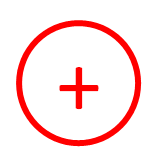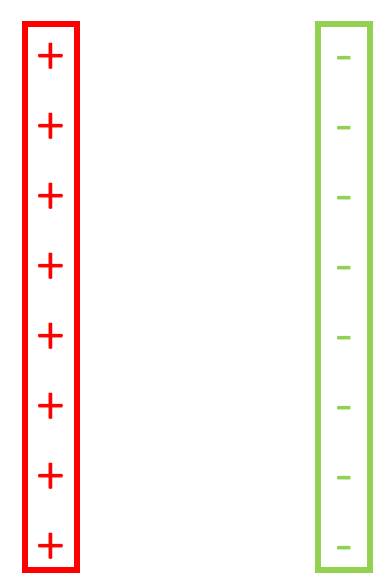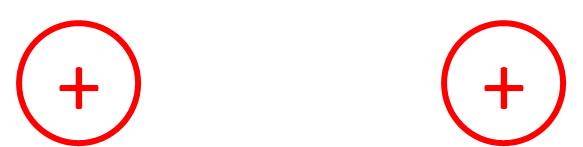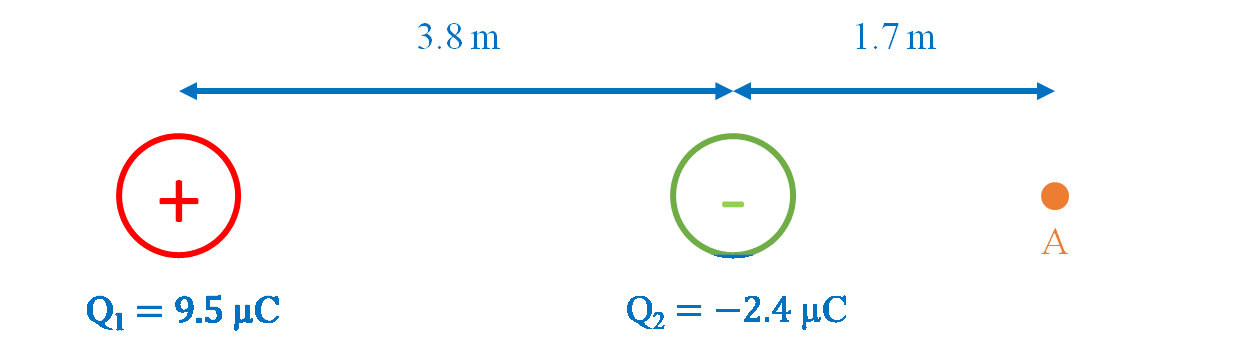# Electric field#### Everything You Need in One Place

Homework problems? Exam preparation? Trying to grasp a concept or just brushing up the basics? Our extensive help & practice library have got you covered.#### Learn and Practice With Ease

Our proven video lessons ease you through problems quickly, and you get tonnes of friendly practice on questions that trip students up on tests and finals.#### Instant and Unlimited Help

Our personalized learning platform enables you to instantly find the exact walkthrough to your specific type of question. Activate unlimited help now!##### Examples
###### Lessons
1. How to draw electric field line diagrams
Draw the electric field line diagrams for the following:
1. Positive point charge2. Negative point charge3. Parallel plates with equal magnitude charges4. Two point charges with opposite equal magnitude charges5. Two point charges with positive equal magnitude point charges6. Two point charges with negative equal magnitude point charges2. Calculations with electric field
A pair of positive and negative point charges are fixed in the following positions:1. Find the electric field (magnitude and direction) at point A.
2. A charge placed at A experiences a force of $5.9 \times 10^{-3} N$ [right]. Find the magnitude and polarity of the charge.
###### Topic Notes
In this lesson, we will learn:
• Meaning of electric field and its relationship to electric force
• How to draw electric field line diagrams
• Solving problems with electric field
Notes:

• Electric field (E) is the electric force exerted by a charge Q on another charge q, per unit charge of q. It is a vector quantity.
• By convention, the direction of electric field vectors is defined as the direction that a positive test charge would move if placed in the field. Fe points in the same direction as E for positive charges, and in the opposite direction of E for negative charges.
• A test charge is a point charge with a very small magnitude. Test charges have a small magnitude charge so that the electric field of the test charge is negligible and does not affect the electric field that is being investigated.

Electric Field

$E= \frac{F_e}{Q}$
or equivalently, by substituting Coulomb's law:
$|E| = k \frac{|Q|}{r^2}$
$E:$ electric field, in newtons per coulomb (N/C)
$F_e:$ electric force, in newtons (N)
$q:$ charge that experiences the field, in coulombs (C)
$k:$ Coulomb's law constant, $9.00 \times 10^9 N\centerdot m^2 / C^2$
$|Q|:$ magnitude of charge that creates the field, in coulombs (C)
$r:$ distance from charge, in meters (m)

Coulomb's Law (Electric Force)

$|F_e| = k \frac{|Q_1 Q_2|}{r^2}$
$|F_e|:$ magnitude of electric force, in newtons (N)
$k:$ Coulomb's law constant, $9.00 \times 10^9 N\centerdot m^2 / C^2$
$|Q_1|, |Q_2|:$ magnitude of each charge, in coulombs (C)
$r:$ distance between charges, in meters (m)